# 1 Introduction 2 2 Evaluation of Functions 3

 Date 28.05.2018 Size 73.7 Kb. #51828

1 Introduction 2

2 Evaluation of Functions 3

3 Compositional (Construct) Operators 4

4 Some Implementation Issues 6

5 Lambda Calculus 11

1. ## Introduction

Imperative (Procedural) Programming Paradigm rely heavily on modifying the values of a collection of variables, called the state. If we neglect the input/output operations and the possibility that a program might run continuously (e.g. the controller for a manufacturing process), we can arrive at the following abstraction. Before execution, the state has some initial value representing the inputs to the program, and when the program has finished, the state has a new value including the result(s). Moreover, during execution, each command changes the state, which has therefore proceeded through some finite sequence of values:

iS = S1 -> S2 -> … -> Sn -> fS

For example in a sorting program, the state initially includes an array of values (iS), and when the program has finished, the state has been modified in such a way that these values are sorted (fS), while the intermediate states represent progress towards this goal.

The state is typically modified by imperative assignment commands, often written in the form

v = E or v := E

where v is a variable and E some expression. These commands can be executed in a sequential manner by writing them one after the other in the program, often separated by a semicolon.

Functional programming represents a radical departure from this model. Essentially, a functional program is simply an expression, and execution means evaluation of the expression.

We can see how this might be possible, in general terms, as follows. Assuming that an imperative program (as a whole) is deterministic, i.e. the output is completely determined by the input, we can say that the final state, or whichever fragments of it are of interest, is some function of the initial state, say fS = f(iS).

In functional programming this view is emphasized: the program is actually an expression that corresponds to the mathematical function f . Functional languages support the construction of such expressions by allowing rather powerful functional constructs.

To illustrate the distinction between imperative and functional programming, the factorial function might be coded imperatively as:

function factorial(n)

{

var x = 1;

while (n > 0)

{

x = x * n;

n = n ­ 1;

}

return x;

}

whereas it would be expressed in the functional paradigm, as a recursive function:

factorial n =

if n = 1 then 1

else n * factorial(n ­ 1);

1. ## Evaluation of Functions

The main distinctive feature of the Functional Programming Paradigm is however a way in which a function is evaluated.

For example, in procedural paradigm, the operator

factorial (3)

always returns an integer value (6 in this particular case).

In accordance with the Functional Paradigm, the expression

factorial (3)

returns a so-called normal form of function “factorial”.

Primitively speaking, a normal form can be produced by replacing of internal functions with their bodies (source texts).

Example:

factorial n =

if n = 1 then 1

else n * factorial(n ­ 1);

factorial(3) = 3* factorial(2) = 3*2*factorial(1) =3*2*1

In other words expression 3*2*1 is a normal form for expression factorial(3).

In more general terms, a functional program consists of an expression E (representing both the algorithm and the input). This expression E is subject to some rewrite rules. Reduction consists of replacing some part P of E by another expression P' according to the given rewrite rules. ... This process of reduction will be repeated until the resulting expression has no more parts that can be rewritten. The expression E* thus obtained is called the normal form of E and constitutes the output of the functional program.

Thus, if we say “function E returns E*”, we mean that E* is a normal form of E.

There is also a special function, eval, which convert expressions to values.

eval(factorial(3)) = eval (3*2*1) = 6

1. ## Compositional (Construct) Operators

Functional programming is based on the mathematical concept of a function and functional programming languages include at least the following:

• Set of data structures and associated functions

• Set of Primitive Functions.

• Set of Composition (Construct) Operators.

Let us illustrate these components by the following example:

Suppose a functional language support a data structure called List as a basic data structure.

Examples of lists: [1,5,6] [‘Nick’, ‘Thomas’, ‘Denis’}

Lists may include other lists as members: [1, [ ‘Nick’, ‘Thomas’, ‘Denis’],2, [25,36,72] ]

Suppose also that nil represents an empty list. nill = []

Additionally, two very simple functions would be useful:

• Function true() returns Boolean value “true” if evaluated

• Function false() returns Boolean value “false” if evaluated

Among the built in (primitive) functions for list manipulation are

• cons for attaching an element as a first one to the list (concatenation),

• car for extracting the first element of a list, and

• cdr which returns a list minus its first element.

Examples:

CONS(‘Palina’ , [‘Nick’, ‘Thomas’, ‘Denis’]) = [‘Palina’, ‘Nick’, ‘Thomas’, ‘Denis’]

CONS([‘Nick’, ‘Thomas’, ‘Denis’], [25,36,72]) = [ [‘Nick’, ‘Thomas’, ‘Denis’], 25,36,72]

CAR([‘Nick’, ‘Thomas’, ‘Denis’]) = ‘Nick’

CDR([‘Nick’, ‘Thomas’, ‘Denis’]) = [ ‘Thomas’, ‘Denis’, ‘Palina’]

CONS(CDR(xList), CAR(xList)) = xList

Suppose, there is also a special operator COND. The operator may be seen as a list which consists of a finite number of pairs of elements. Each pair includes a condition and another function. The function that is used as first parameter, is evaluated and if it returns the Boolean value "TRUE", the second parameter replaces the COND operator.

The operator COND may be seen in the following form:

 COND{ {, } {, } . . . . . . {, } }

The function "function_k" replaces the operator COND, if

1. evaluation of "condition_k" returns the boolean value "TRUE" ;

2. all previous conditions (i.e., conditions , , ..., ) return the boolean value "FALSE".

The operator COND is an example of a compositional (construct) operator

A new function CHECK(list, element) that returns (has a normal form as)

• true() if the element is a member of the list

• false() otherwise

can be defined as follows:
CHECK list, element

COND

{

{list = nill, false ()}

{CAR(list) = element, true()}

{true(), CHECK(CDR(list),element)}

}

Let us see how a normal form of function CHECK([bca],a) is inferred:

CHECK([bca],a) = CHECK([ca],a) = CHECK([a],a) = true()
Conditional construct:

 If then else

Is another example of a compositional (construct) operator.

Example:

CHECK list, element

If (list = nill) false()

else

{

if (CAR(list) = element) true()

else CHECK(CDR(list),element)

}

1. ## List Data Types

A data type “List” is rather important for all programming languages based on Functional Paradigm.

For example, list [1,2,3] can be implemented as follows: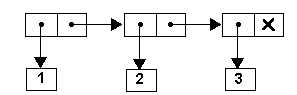Analogically, the list [1,[‘Nick’,’Alex’],3] can be seen as follows: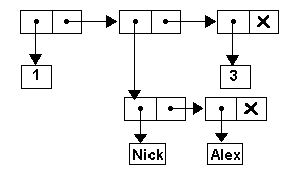1. ## Presenting Functions as Lists

It is interesting, that functions are presented in the same form of a list. First element of such list presentation is called a head, and refers to the function body in the same way as other elements refer (or may refer) to corresponding values.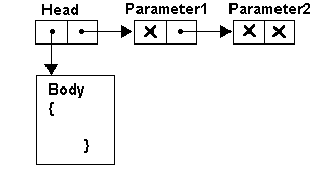Consider the previously mentioned function

factorial n =

if n = 1 then 1

else n * factorial(n ­ 1);

Recollect that the function is subject to some rewrite rules which are defined by the function body.

The process of reduction of the function factorial(3) is illustrated by the following diagram: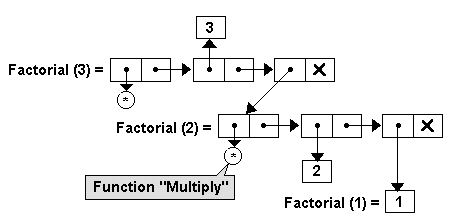Using prexix notation, the list can be wtitten as:

[*,3,[*,2,1]]

Obviously, result of evalauation of the function factorial(3) is a single value 6.

We have said that from a theoretical point of view, reduction of expressions is a basic property of Functional Paradigm. However it has some practical defects. For example, consider the following two functions:

factorial n =

if n = 1 then 1

else n * factorial(n ­ 1);

--------------------------------

triple x =

x + x + x

A normal reduction of the function triple(factorial(3)) results in

[+,[*,3,[*,2,1]], [*,3,[*,2,1]], [*,3,[*,2,1]] ]

and the system needs to evaluate three separate instances of the same expression [*,3,[*,2,1]]. This is unacceptable in practice.

Another problem can be easily detected if we define a new function sumUp which is supposed to be applied to a list of arithmetical values and simply sums up all the values of the list.

sumUp list =

COND

{

{list = nill, 0}

{true(),CAR(list) + sumUp(CDR(list))}

}

Reduction of the function sumUp() results in [+,6,0]. At the same time, reduction of the function sumUp(factorial(3)) might result in run-time error because the function sumUp will be applied to a list looking as follows:

[*,3,[*,2,1]]

and will result into a normal form

[+,*,sumUp(3,[*,2,1])]

which cannot be evaluated in a meningful way.

1. ## Lazy and Eager Evaluations

There are two main solutions to this problem, and these solutions divide the world of functional programming languages into two camps.

The first alternative is to stick with normal order reduction, but attempt to optimize the implementation so multiple subexpressions arising in this way are shared, and never evaluated more than once. Internally, expressions are represented as directed acyclic graphs rather than as trees. This is known as lazy or call­by­need evaluation, since expressions are only evaluated when absolutely necessary.

For exanple, the reduction of function triple(factorial(3)) may look as follows: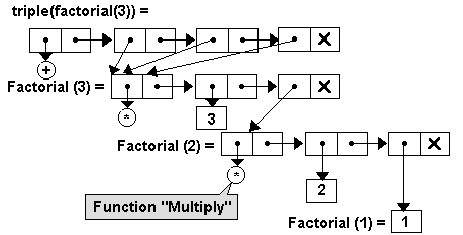The second approach is to turn the theoretical considerations about reduction strategy on their head and evaluate arguments to functions before passing the values to the function. This is known as applicative order or eager evaluation.

The latter name arises because function arguments are evaluated even when they may be unnecessary. Of course, eager evaluation means that some expressions may loop that would terminate in a lazy regime. But this is considered acceptable, since these instances are generally easy to avoid in practice.

Laziness leads to some elegant solutions for problems involving infinite series and seems more consistent with the idea of functional programming.

However, because the order in which functions are used is not very clear to the programmer, the speed and efficiency of programs written in lazy languages can be difficult to understand.

1. ## Controlling Evaluation

The fact that functions are not automatically evaluated is of crucial importance to advanced programmers. It gives precise control over the evaluation of expressions, and can be used to mimic many of the helpful cases of lazy/eager evaluation. We shall see a very simple instance in the next section.

There is also a special function, eval, which convert expressions to values.

The use of “eval” allows us to make additional features primitive, with their own special reduction procedures, rather than implementing them directly in terms of functions.

Example:

Suppose we are going to use the function CHECK defined above as:
CHECK list, element

COND

{

{list = nill, false ()}

{CAR(list) = element, true()}

{true(), CHECK(CDR(list),element)}

}

in the body of new function ConditionalCONS which adds just unique elements to a list and skips duplicate elements:

ConditionalCONS list, element

COND

{

{eval(CHECK (list,element)), list}

{true(), CONS(list,element)}

}
here we need explicitly evaluate the function CHECK to convert the expression to a Boolean value as requested by the COND construct. Actually, we modify the usual reduction strategy so that first the boolean expression is evaluated, and only then is exactly one of the two arms evaluated, as appropriate. Otherwise the system would attempt to make reduction of CONS(list,element) event if it is not necessary.

The function quote is in a certain sense opposite to the function eval and explicitly returns a parameter as an expression, i.e. “quote” tells the interpreter not to evaluate the argument inside it.

Example:

Note that the function ConditionalCONS(list, element) returns a list of objects which generally cannot be evaluated.
ConditionalCONS([‘Nick’,’Alex’],’Nick’) = [‘Nick’,’Alex’]

ConditionalCONS([‘Nick’,’Alex’],’Palina’) = [‘Palina’,‘Nick’,’Alex’]

Suppose, we have defined also the function getNumberOfListElements as:
getNumberOfListElements list

COND

{

{list = nill, 0}

{true(), 1 + getNumberOfListElements (CDR(list))}

}

In case of eager evaluation, the combination of these two functions should be defined using the “quote” function.

GetNumberOfListElements (quote(ConditionalCONS(list,element)));

In case of lazy evaluation, the function:

GetNumberOfListElements (ConditionalCONS(([‘Nick’,’Alex’],’Palina’))

returns normal form [+,1,[+,1,1]]

the function:

eval(GetNumberOfListElements (ConditionalCONS(([‘Nick’,’Alex’],’Palina’)))

returns just value “3”.

1. ## Lambda Calculus

The Lambda-calculus is a universal model of computation, that is, any computation that can be expressed in a Turing machine can also be expressed in the lambda calculus. Functional Programming Paradigm is based on the Lambda Calculus. Moreover, it is said that Functional Programming Paradigm is just an implementation of Lambda Calculus.
1. ## Functions and Lambda Notation

A function accepts input and produces an output. Suppose we have a "goodGuy(x)" function that produces the following reult: “x” is a good guy

Obviously the goodGuy function produces the following outputs for the corresponding inputs:

Nick -> Nick is a good guy

Alex -> Alex is a good guy

We can use Lambda-calculus to describe such a function as:

Lx.goodGuy x

This is called a lambda-expression or abstraction (Here the "L" is supposed to be a lowercase Greek "lambda" character).

If we want to apply the function to an argument, we use the following syntax:

(Lx.goodGuy x)Alex

This notation is known as application of an abstraction (or simply application).

The application (Lx.goodGuy x)Alex returns ‘Alex is a good guy’

Functions can also be the result of applying a lambda-expression, as with this Guy(x,y) function that produces the following result: “x” is a “y” guy

Ly.Lx.Guy x y

We can use application of this abstraction to create a goodGuy abstraction:

(Ly.Lx.Guy x y)good -> Lx.goodGuy x

Functions can also be the inputs to other functions, as with this "getNameByPhone" function:

Lp.getNameByPhone p

We can feed the getNameByPhone function to the "goodGuy" function:

(Lx.goodGuy x) (Lp.getNameByPhone p)5831618

Formal Lambda Calculus

Lambda-expressions have the following syntax:

lambda-expression ::= variable

| constant

| application

| abstraction

application ::= (lambda-expression)lambda-expression

abstraction ::= Lvariable.lambda-expression

The evaluation of lambda-expression is from the application of two reduction rules.

• The alpha-reduction rule says that we can consistently rename bindings of variables:

Lx.E -> Lz.{z/x}E

where {z/x}E means the substitution of z for x for any occurrence of x in E.

• The beta-reduction rule says that application of a lambda-expression to an argument is the consistent replacement of the argument for the lambda-expression's bound variable in its body:

(Lx.P)Q -> [Q/x]P

where [Q/x]P means the substitution of Q for x for any free occurrence of x in P.

The Church-Rosser Theorem states that the final result of a chain of substitutions does not depend on the order in which the substitutions are performed.

1. ## Universality of Lambda-Calculus

As it was already mentioned, the Lambda-calculus is a universal model of computation . To show this, here is the translation of a conditional control structure into lambda-calculus:

We can define three lambda abstractions as follows:

true = Lx.Ly.x

false = Lx.Ly.y

if-then-else = La.Lb.Lc.((a)b)c

Consider the following Lambda application

(((if-then-else)false)Nick)Palina

meaning something like

if (false)

then Nick

else Palina

Obviously, we would expect a result of reduction as ‘Palina’.

Let us see how the reduction rules can be applied

(((if-then-else)false)Nick)Palina =

(((La.Lb.Lc.((a)b)c)Lx.Ly.y)Nick)Palina = /*a -> false -> Lx.Ly.y */

((Lb.Lc.((Lx.Ly.y)b)c)Nick)Palina = /*b -> ‘Nick’ */

(Lc.((Lx.Ly.y)Nick)c)Palina = /*c -> ‘Palina’ */

((Lx.Ly.y)Nick)Palina = /*x -> ‘Nick’ */

(Ly.y) Palina = /*y -> ‘Palina’ */

Palina

You wouldn't want to really program like this, though.

1. ## From Theory to Programming Language

Although the lambda-calculus is powerful enough to express any program, this doesn't mean that you'd actually want to do so. After all, the Turing Machine offers an equally powerful computational basis.

The strength of the lambda-calculus is that it is easily used as a "glue" on top of a richer world of primitives. Its advantages as a glue are that it has a natural correspondence with the way that people program, and natural compilation techniques yield high-performance code. The latter comes through optimizations know as tail-call and continuation-passing, which might be the subject of future talks.

There are software engineering advantages to a language glued together with lambda-calculus. Lambda expressions can be understood locally - their dependence on their environment is entirely through their free variables. Lambda expressions tend to have fewer free variables and more bound variables than comparable imperative code, since they do not rely as heavily on assignment to express the computation. An imperative program proceeds by altering some globally-accessible store of values. By contrast, a functional program proceeds by function application and the return of values. This eliminates large classes of errors associated with maintaining a global store of values.